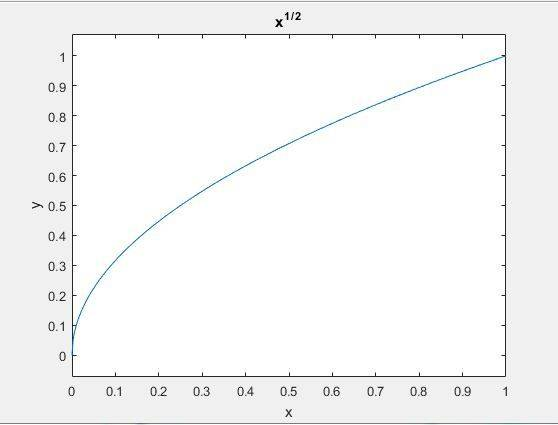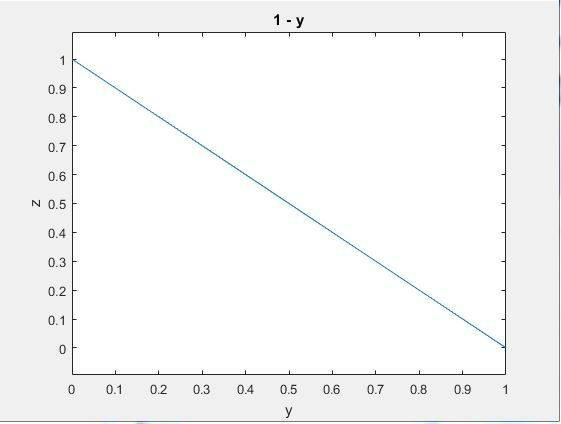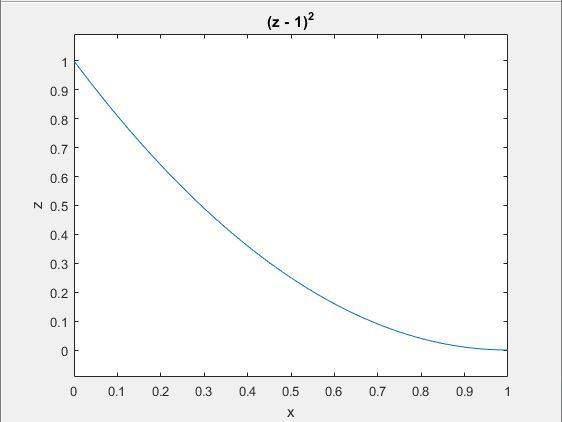# Upper and lower bounds of a triple integral

• TheSodesa

## Homework Statement

Let ##T \subset R^3## be a set delimited by the coordinate planes and the surfaces ##y = \sqrt{x}## and ##z = 1-y## in the first octant.

Write the intgeral
$$\iiint_T f(x,y,z)dV$$
as iterated integrals in at least 3 different ways.

## Homework Equations

$$\iiint_T f(x,y,z)dV = \int_a^b \int_{y_1 (x)}^{y_2 (x)} \int_{z_1 (x,y)}^{z_2 (x,y)} f(x,y,z) dx dy dz$$

## The Attempt at a Solution

My interpretation of this problem is that they want me to "simply" find out the upper and lower bounds of integration for 3 different cases, since there is no specific function to integrate.

This is a bit difficult to explain, since I can't show you a picture of the volume because I can't seem to be able to draw the ##y = \sqrt{x}## correctly in Matlab using this code:

syms x y z

f(x,z) = sqrt(x);
g(x,y) = 1 - y;

hold on
xlabel('x')
ylabel('y')
zlabel('z')
axis equal

ezsurf(f,0:1);
ezsurf(g,0:1)

Anyways, I'm having trouble seeing what the upper bounds are for the innermost iteration, whichever way I try and integrate this thing. For example, if I were to integrate first with respect to y, then x and finally z, I would write:
$$\int_0^1 \int_{0}^{(-z+1)^2} \int_{0}^{?} f(x,y,z) dy dx dz$$
Just looking at the horrible picture that I drew on paper (which kind of looks like a tetrahedron cut off by the ##\sqrt{x}##-surface) is not helping. Does anybody have any hints on where to start. My brain is telling me that ##?## should be a plane of some sort, but the fact that it's cut off by the ##\sqrt{x}## is really throwing me off.

EDIT: The shape is simple (as in x-, y- and z-simple) in all 3 dimensions, so I was able to print out the projections to the different coordinate planes:

y(x):z(y)x(z):Last edited:
$$\int_0^1 \int_{0}^{(1-z)^2} \int_{\sqrt{x}}^{1} f(x,y,z) dy dx dz$$
I'm saying this, because
\begin{cases}

y = \sqrt{x} \iff x = y^2\\
y+z = 1 \iff z = 1- \sqrt{x} \iff x = (1-z)^2
\end{cases}

How does this look?

Last edited:
Using the same logic as above for two other cases:

##dxdydz##:

$$\int_{0}^{1} \int_{0}^{1-z} \int_{0}^{y^2}f(x,y,z) dxdydz$$
and ##dzdxdy##:
$$\int_{0}^{1} \int_{0}^{y^2} \int_{0}^{1-y} f(x,y,z) dzdxdy$$

Last edited: